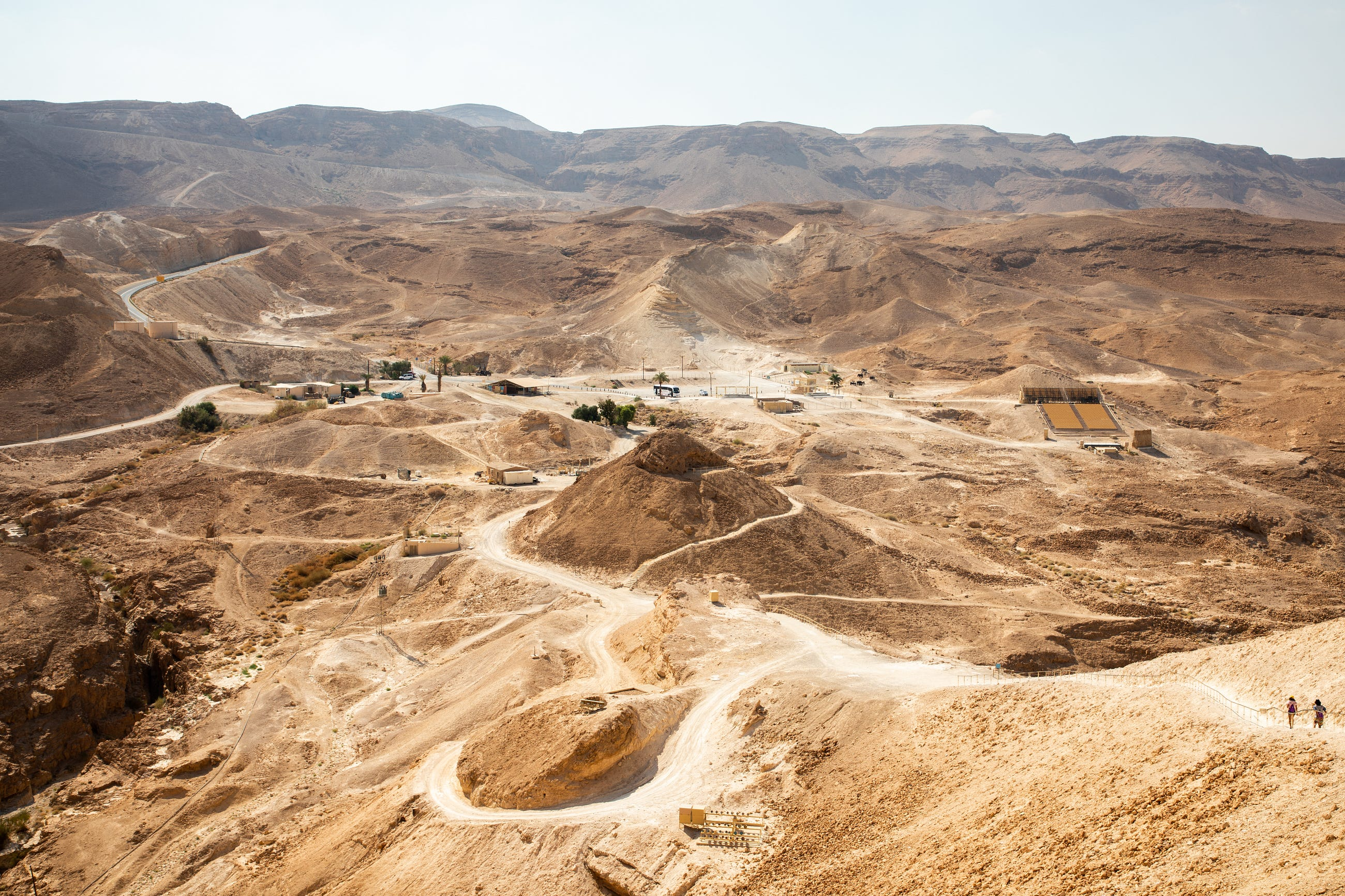# What is Stationarity in Spatial Data?# Case Study — Python Codes

let’s say in “X” field , we have a Silver (Ag) distribution as follows.

`import pandas as pddf = pd.read_csv(‘contoh.csv’)df = df[[‘ID’,’XCOO’,’YCOO’,’Ag’]]`

ID is the identifier of each sample taken from the field, while XCOO and YCOO are X and Y coordinates respectively. To recognize the variation of our data, we visualize it in ID vs AG plot using `pyplot` as figure 2 below:

`import matplotlib.pyplot as pltplt.figure()plt.plot(df.ID,df.Ag,color=’k’)plt.title(‘Ag Field Data’)plt.xlabel(‘ID’)plt.ylabel(‘Ag’)plt.show()`

## Trend Plot

The stationarity of the data could be analyzed using a trend plot, we can simply calculate the intercept and slope of the linear regression equation using `scipy`

`from scipy import statsslope, intercept, corrcoeff, p_value, stderr = stats.linregress(df.ID,df.Ag)m = slopec = intercept`

By extracting slope and intercept from `linregress` we can define and plot our linear function (y = mx + c) as shown at Figure 3.

`#linear regression functiondf.Y = m * df.ID + cplt.figure()plt.plot(df.ID, df.Ag, color=’k’) #point plottingplt.plot(df.ID, df.Y, linewidth=3.5, color=’y’, label=’trend’) #trend plottingplt.title(‘Ag Field Data’)plt.xlabel(‘ID’)plt.ylabel(‘Ag’)plt.legend()plt.show()`

We can see that the trend shown in Figure 3 is a straight horizontal line which indicates that our data is stationary. But is it really stationary? let’s check the trend by partitioning this data (for example on ID 650–750). By using the same code as above, we can visualize the result as in Figure 4.

Figure 4 shows one of the stationarity characteristics. “The stationarity assessment depends on scale”. According to the trend plots, it can be seen that the second plot from ID 650–750 is not stationary despite the result from the whole data appears to be stationary. This stationarity uncertainty also applies whether we take another scale / partition on our data.

## Variogram Plot

In terms of the stationarity assessment, variogram plot has a different approach compare to trend plot. Variogram is a geostatistical method that can visualize variance trend on spatial (lag from one data to another) perspective . Variogram is a highly recommended way to analyze uncertainty since it can see the pattern of relationships between data points at each lag.

Figure 5 shows the Variogram plot consisting of data point (black point) and modelled / theoretical variogram (black line). We also can see other important variables such as:

• Lag distance: The distance between pairs calculated by experimental variogram. e.g: lag distance can be calculated every 10m, 20m,1000m, etc.
• Semivariance/variance: a parameter that describes the dissimilarity between data. The higher the semivariance / variance, the worse the similarity relationship between the data.
• Sill: Variogram value when it reaches a constant point
• Range: Lag distance when the variogram value reaches sill
• Nugget: the variogram “jump” at the starting point (lag distance is ~ 0)

Stationary data can be indicated from a bounded variogram, while non-stationary data can be indicated from an unbounded variogram, as shown in Figure 6.

Bounded variogram is a variogram that has sill & range, while unbounded variogram is a variogram that has an upward trend and does not have sill & range (unflatted).

Now let’s create variogram using our data with `geostatspy` library. `geostatspy` is a python library developed by MichaelPyrcz (@geostatsguy) which contains modules related to geostatistics. You can simply install the library by using `pip install geostatspy` .

In order to plot the experimental variogram we will plot data point in every 7000 lag with 3000 lag tolerance with n = 30. The azimuth is 0 degree with 90 degree tolerance as the illustration shown in Figure 7.

`lag = 7000lag_tol = 3000nlag = 30azi = 0azi_tol = 90bandwidth = 9999lags, gammas, npps = geostats.gamv(df,”XCOO”,”YCOO”,”Ag”,-9999,9999,lag,lag_tol,nlag,azi,azi_tol,bandwidth,isill=1.0)`

`gammas` obtained from `geostats.gamv` is the variogram value for each lag that we determined before, while `npps` is the number of data pairs at a specified lag. After that we can plot `lags` vs `gammas` using `pyplot` as Figure 8 shows.

`# plot experimental variogramplt.figure() plt.scatter(lags,gammas,color = ‘black’,s = npps*0.1,label = ‘Azimuth ‘ +str(azi))plt.legend()plt.xlabel(‘Lag Distance’)plt.ylabel(‘Variogram’)plt.title(‘Ag Distribution Variogram’)plt.xlim([0,175000]); plt.ylim([0,1.5])plt.grid()plt.show()`

Thenceforth we need to plot our modelled variogram to analyze the stationarity in our data. Basically this step needs a “trial and error” to make sure our model fit with our experimental variogram. In this article we already got our best parameter for our modelled variogram.

Sill: 1 | Range: 110000 | Type: Spherical

By using `gslib.make_variogram` and `geostats.vmodel` module we can extract the function of our modelled variogram.

`#variogram modellingnug = 0.5nst = 2 #whether a normal score transformation is used on this variogram (two (2) is for not using)it1 = 1 #variogram type (one (1) is for spherical type)cc1 = 0.5 #sill minus nuggetazi1 = azi #azimuthhmaj1 = 110000 #maximum major lag distance measured in this variogram (range)hmin1 = 110000 #considering not using a minor azimuth (we can ignore this parameter)#assumption no contribution on minor structure (we can ignore this)it2 = 1; cc2 = 0; azi2 = 0; hmaj2 = 9999.9; hmin2 = 400 xlag=15000#make model objectvario = gslib.make_variogram(nug,nst,it1,cc1,azi1,hmaj1,hmin1,it2,cc2,azi2,hmaj2,hmin2)#extract the important variable of the modelled variogramindex_maj,h_maj,gam_maj,cov_maj,ro_maj = geostats.vmodel(nlag,xlag,azi,vario)`

Then we overlay our modelled variogram into our previous experimental variogram plot as shown in Figure 9.

`plt.plot(h_maj,gam_maj,color = ‘black’)`

From Figure 9 it can be seen that the variogram modelled fit with the experimental variogram. This variogram is categorized as bounded variogram considering the sill / range which is very clear. It can be decided that our data tends to be stationary.